Analysis by: Clive Fuentebella

ALIASES:

Gen:Variant.Razy.548673 (BITDEFENDER)

PLATFORM:

Windows

OVERALL RISK RATING: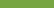DAMAGE POTENTIAL: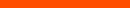DISTRIBUTION POTENTIAL:REPORTED INFECTION:INFORMATION EXPOSURE: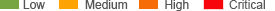•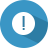Threat Type: Ransomware
•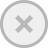Destructiveness: No
•Encrypted: No
•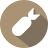In the wild: Yes

## OVERVIEW

This Ransomware arrives on a system as a file dropped by other malware or as a file downloaded unknowingly by users when visiting malicious sites.

It encrypts files with specific file extensions. It encrypts files found in specific folders.

## TECHNICAL DETAILS

File Size: 389,120 bytes
File Type: EXE
Memory Resident: Yes
Initial Samples Received Date: 01 Oct 2019
Payload: Displays message/message boxes, Encrypts files

Arrival Details

This Ransomware arrives on a system as a file dropped by other malware or as a file downloaded unknowingly by users when visiting malicious sites.

Installation

This Ransomware drops the following copies of itself into the affected system:

• %Application Data%\sysem.exe

(Note: %Application Data% is the current user's Application Data folder, which is usually C:\Documents and Settings\{user name}\Application Data on Windows 2000(32-bit), XP, and Server 2003(32-bit), or C:\Users\{user name}\AppData\Roaming on Windows Vista, 7, 8, 8.1, 2008(64-bit), 2012(64-bit) and 10(64-bit).)

• "%System%\cmd.exe" /c copy /y "{Malware file path and name}" "%Application Data%\sysem.exe"
• "{Malware file path and name}" runas
• "%Application Data%\sysem.exe"
• mshta.exe "javascript:o=new ActiveXObject('Scripting.FileSystemObject');setInterval(function(){try{o.DeleteFile('{Malware name}');close()}catch(e){}}10);"
• mshta.exe "javascript:o=new ActiveXObject('WScript.Shell');x=new ActiveXObject('Scripting.FileSystemObject');setInterval(function(){try{i=x.GetFile('sysem.exe').Path;o.RegWrite('HKCU\Software\Microsoft\Windows\CurrentVersion\RunOnce\hetvuEz'i);}catch(e){}}10);";
• mshta.exe "javascript:eval(new ActiveXObject('WScript.Shell').RegRead('HKCU\Software\{5 random characters}\{5 random characters}'));close();";
• "%System%\cmd.exe" /c wbadmin DELETE SYSTEMSTATEBACKUP -keepVersions:0
• "%System%\cmd.exe" /c wmic SHADOWCOPY DELETE
• "%System%\cmd.exe" /c bcdedit /set {default} recoveryenabled No
• "%System%\cmd.exe" /c bcdedit /set {default} bootstatuspolicy ignoreallfailures

(Note: %Application Data% is the current user's Application Data folder, which is usually C:\Documents and Settings\{user name}\Application Data on Windows 2000(32-bit), XP, and Server 2003(32-bit), or C:\Users\{user name}\AppData\Roaming on Windows Vista, 7, 8, 8.1, 2008(64-bit), 2012(64-bit) and 10(64-bit).)

Autostart Technique

This Ransomware adds the following registry entries to enable its automatic execution at every system startup:

HKEY_CURRENT_USER\Software\Microsoft\
Windows\CurrentVersion\RunOnce
hetvuEz = %Application Data%\sysem.exe

Other Details

This Ransomware adds the following registry keys:

HKEY_CURRENT_USER\Software\hetvuEz

HKEY_CURRENT_USER\Software\{5 random characters}

Ransomware Routine

This Ransomware encrypts files with the following extensions:

• 000
• 001
• 1
• 101
• 103
• 108
• 110
• 123
• 128
• 1cd
• 1sp
• 1st
• 3
• 3d
• 3d4
• 3dd
• 3df
• 3df8
• 3dm
• 3dr
• 3ds
• 3dxml
• 3fr
• 3g2
• 3ga
• 3gp
• 3gp2
• 3mm
• 3pr
• 3w
• 4w7
• 602
• 7z
• 7zip
• 8
• 89t
• 89y
• 8ba
• 8bc
• 8be
• 8bf
• 8bi8
• 8bl
• 8bs
• 8bx
• 8by
• 8li
• 8svx
• 8xt
• 9xt
• 9xy
• a\$v
• a2c
• aa
• aa3
• aac
• aaf
• aah
• aaui
• ab4
• ab65
• abc
• abk
• abt
• abw
• ac2
• ac3
• ac5
• acc
• accdb
• accde
• accdr
• accdt
• ace
• acf
• ach
• acp
• acr
• acrobatsecuritysettings
• acrodata
• acroplugin
• acrypt
• act
• aea
• aec
• aep
• aepx
• aes
• aet
• afdesign
• afm
• afp
• agd1
• agdl
• age3rec
• age3sav
• age3scn
• age3xrec
• age3xsav
• age3xscn
• age3yrec
• age3ysav
• age3yscn
• ahf
• ai
• aif
• aiff
• aim
• aip
• ais
• ait
• ak
• al
• al8
• ala
• alb3
• alb4
• alb5
• alb6
• ald
• ali
• allet
• alt3
• alt5
• amf
• aml
• amr
• amt
• amu
• amx
• amxx
• anl
• ann
• ans
• ansr
• anx
• aoi
• ap
• apa
• apd
• ape
• apf
• api
• apj
• apk
• apnx
• apo
• app
• approj
• apr
• apt
• apw
• apxl
• arc
• arch00
• arff
• ari
• arj
• aro
• arr
• ars
• arw
• as
• as\$
• as3
• asa
• asc
• ascm
• ascx
• asd
• ase
• asf
• ashx
• asl
• asm
• asmx
• asn
• asnd
• asp
• aspx
• asr
• asset
• ast
• asv
• asvx
• asx
• ath
• atl
• atomsvc
• atw
• automaticdestinations-ms
• aux
• av
• avi
• avn
• avs
• awd
• awe
• awg
• awp
• aws
• awt
• aww
• awwp
• ax
• azf
• azs
• azw
• azw1
• azw3
• azw4
• b
• b27
• b2a
• back
• backup
• backupdb
• bak
• bak~
• bamboopaper
• bank
• bar
• bau
• bax
• bay
• bbcd
• bbl
• bbprojectd
• bbs
• bbxt
• bc5
• bc6
• bc7
• bcd
• bck
• bcp
• bdb
• bdb2
• bdp
• bdr
• bdt2
• bdt3
• bean
• bfa
• bgt
• bgv
• bi8
• bib
• bibtex
• bic
• big
• bik
• bil
• bin
• bina
• bizdocument
• bjl
• bk
• bk!
• bk1
• bk2
• bk3
• bk4
• bk5
• bk6
• bk7
• bk8
• bk9
• bkf
• bkg
• bkp
• bks
• bkup
• bld
• blend
• blend2
• blg
• blk
• blm
• blob
• blp
• bmc
• bmf
• bmk
• bml
• bmm
• bmml
• bmp
• bmpr
• bna
• boc
• book
• bop
• bp1
• bp2
• bp3
• bpf
• bpk
• bpl
• bpm
• bpmc
• bps
• bpw
• brd
• brh
• brl
• brs
• brx
• bsa
• bsk
• bso
• bsp
• bst
• btd
• btf
• btoa
• btx
• burn
• burntheme
• bvd
• bwd
• bwf
• bwp
• bxx
• bzabw
• c
• c2e
• c6
• cae
• cag
• calca
• cam
• camproj
• cap
• capt
• car
• caro
• cas
• cat
• catproduct
• cawr
• cbf
• cbor
• cbr
• cbz
• cc
• ccc
• ccd
• ccf
• cch
• ccitt
• cd
• cd1
• cd2
• cdc
• cdd
• cddz
• cdf
• cdi
• cdk
• cdl
• cdm
• cdml
• cdmm
• cdmz
• cdpz
• cdr
• cdr3
• cdr4
• cdr5
• cdr6
• cdrw
• cds
• cdt
• cdtx
• cdx
• cdxml
• ce1
• ce2
• cef
• cer
• cert
• cf5
• cfd
• cfg
• cfp
• cfr
• cgf
• cgfiletypetest
• cgi
• cgm
• cgp
• chi
• chk
• chm
• chml
• chmprj
• chp
• chpscrap
• cht
• chtml
• cib
• cida
• cif
• cipo
• civ4worldbuildersave
• civbeyondswordsave
• cl2arc
• cl2doc
• clam
• clarify
• class
• clb
• clkd
• clkt
• clp
• clr
• cls
• clx
• cmf
• cml
• cmp
• cms
• cmt
• cmu
• cnf
• cng
• cnt
• cnv
• cod
• col
• comicdoc
• comiclife
• compositionmodel
• compositiontemplate
• con
• conf
• config
• contact
• converterx
• cp
• cpc
• cpd
• cpdt
• cphd
• cpi
• cpio
• cpp
• cpy
• cr2
• crashed
• craw
• crb
• crd
• creole
• cri
• crjoker
• crs
• crs3
• crt
• crtr
• crw
• crwl
• crypt
• crypted
• cryptowall
• cryptra
• cs
• cs8
• csa
• cse
• csh
• csi
• csl
• cso
• csp
• csr
• css
• cst
• csv
• ctbl
• ctd
• cte
• ctf
• ctl
• ctt
• ctxt
• cty
• cue
• current
• cvj
• cvl
• cvw
• cw3
• cwf
• cwk
• cwn
• cwr
• cws
• cwwp
• cyi
• cys
• d
• d3dbsp
• dac
• dag
• dal
• dap
• das
• dash
• dat
• database
• datx
• dayzprofile
• dazip
• db
• db_journal
• db0
• db3
• dba
• dbb
• dbc
• dbf
• dbfv
• db-journal
• dbk
• dbr
• dbs
• dbx
• dc2
• dc4
• dca
• dcd
• dcf
• dch
• dco
• dcp
• dcr
• dcs
• dct5
• dcu
• ddc
• ddcx
• ddd
• ddif
• ddoc
• ddrw
• dds
• deb
• debian
• dec
• ded
• default
• del
• dem
• der
• des
• desc
• description
• design
• det
• deu
• dev
• dex
• dfe
• dfl
• dfm
• dft
• dfti
• dgc
• dgm
• dgpd
• dgr
• dgrh
• dgs
• dhe
• dic
• did
• dif
• dii
• dim
• dime
• dip
• dir
• directory
• disc
• disco
• disk
• dit
• divx
• diz
• djbz
• djv
• djvu
• dk@p
• dlc
• dlg
• dmbk
• dmg
• dmp
• dmtemplate
• dmv
• dna
• dng
• dnl
• dob
• doc
• doc#
• docb
• doce
• docenx
• dochtml
• docl
• docm
• docmhtml
• docs
• docset
• docstates
• doct
• documentrevisions-v100
• docx
• docxl
• docxml
• dok
• dot
• dothtml
• dotm
• dotmenx
• dotx
• dotxenx
• dox
• doxy
• doz
• dp
• dpd
• dpi
• dpk
• dpl
• dpr
• drd
• dream
• drf
• drm
• drmx
• drmz
• drw
• dsc
• dsd
• dsdic
• dsf
• dsg
• dsk
• dsl
• dsn
• dsp
• dsy
• dtd
• dtm
• dtml
• dtp
• dtx
• dump
• dvb
• dvd
• dvi
• dvs
• dvx
• dvz
• dwd
• dwdoc
• dwf
• dwfx
• dwg
• dwlibrary
• dwp
• dwt
• dxb
• dxd
• dxe
• dxf
• dxg
• dxn
• dxr
• dxstudio
• dzp
• e3s
• e4a
• easmx
• ebk
• ebs
• ec4
• ecc
• ecr
• edb
• edd
• edf
• edl
• edml
• edn
• edoc
• edrwx
• edt
• edz
• efa
• efax
• eff
• efl
• efm
• efr
• eftx
• efu
• efx
• egr
• egt
• ehp
• eif
• eip
• ekm
• el6
• eld
• elf
• elfo
• eln
• emc
• emf
• eml
• emlxpart
• emm
• enc
• enciphered
• encrypted
• enfpack
• ent
• enx
• enyd
• eob
• eot
• ep
• epdf
• epf
• epk
• eprtx
• eps
• epsf
• ept
• epub
• eql
• erbsql
• erd
• ere
• erf
• err
• es
• es3
• esc
• esd
• esf
• esm
• esp
• ess
• esv
• et
• ete
• etng
• etnt
• ets
• etx
• euc
• evo
• evy
• ewl
• ex
• exc
• exd
• exf
• exif
• exprwdhtml
• exprwdxml
• exx
• ez
• ezc
• ezm
• ezs
• ezz
• f4v
• f90
• f96
• fac
• fae
• faq
• fax
• fbd
• fbp6
• fbs
• fcd
• fcf
• fcstd
• fd
• fdb
• fdf
• fdoc
• fdr
• fds
• fdseq
• fdw
• fdx
• fed
• feed-ms
• feedsdb-ms
• ff
• ffa
• ffd
• ffdata
• fff
• ffl
• ffo
• fft
• ffx
• fh
• fhd
• fig
• fin
• fl
• fla
• flac
• flag
• flat
• flf
• flib
• flka
• flkb
• flm
• flp
• fls
• flt
• fltr
• flv
• flvv
• fly
• fm
• fm3
• fmc
• fmd
• fmf
• fml
• fmp
• fmp3
• fnf
• fo
• fodg
• fodp
• fods
• fodt
• folio
• for
• forge
• fos
• fountain
• fp
• fpage
• fpdoclib
• fpenc
• fphomeop
• fpk
• fpp
• fpt
• fpx
• fra
• frag
• frdat
• frdoc
• freepp
• frelf
• frm
• fs
• fsc
• fsd
• fsf
• fsh
• fsp
• fss
• ft10
• ft11
• ft7
• ft8
• ft9
• ftil
• ftr
• fwk
• fwtemplate
• fxd
• fxg
• fxo
• fxr
• fzh
• fzip
• ga3
• gam
• gan
• gcsx
• gct
• gdb
• gdc
• gdoc
• ged
• gev
• gevl
• gfe
• gform
• gfx
• ggb
• ghe
• gho
• gif
• gil
• giw
• glk
• glo
• glos
• gly
• gml
• gmp
• gnd
• gno
• gofin
• gp4
• gpd
• gpf
• gpg
• gpn
• gpx
• gpz
• gra
• gray
• grey
• grf
• grk
• grle
• groups
• gry
• gs
• gsa
• gsf
• gsheet
• gslides
• gsm
• gthr
• gui
• gul
• gvi
• gxk
• gxl
• gz
• gzig
• gzip
• h
• h1q
• h1s
• h1w
• h2o
• h3m
• h4r
• haml
• hbk
• hbl
• hbx
• hcl
• hcw
• hda
• hdd
• hdl
• hdt
• hdx
• hed
• help
• helpindex
• hex
• hfd
• hft
• hhs
• hkdb
• hkx
• hlf
• hlp
• hlx
• hlx2
• hlz
• hm2
• hmskin
• hnd
• hoi4
• hot
• hp2
• hpd
• hpj
• hplg
• hpo
• hpp
• hps
• hpt
• hpw
• hqx
• hrx
• hs
• hsm
• hsx
• hta
• htm
• htm~
• html
• htmls
• htmlz
• htms
• htpasswd
• htz5
• hvpl
• hw3
• hwp
• hwpml
• hwt
• hxe
• hxi
• hxq
• hxr
• hxs
• hyp
• hype
• iab
• iaf
• ial
• ibank
• ibcd
• ibd
• ibk
• ibz
• icalevent
• icaltodo
• icc
• icml
• icmt
• ico
• ics
• icst
• icxs
• idap
• idc
• idd
• idl
• idml
• idp
• idx
• ie5
• ie6
• ie7
• ie8
• ie9
• iff
• ifp
• ign
• igr
• ihf
• ihp
• iif
• iiq
• iks
• ila
• ildoc
• img
• imp
• imr
• incp
• incpas
• ind
• indb
• indd
• indl
• indp
• indt
• inf
• info
• ink
• inld
• inlk
• inp
• inprogress
• inrs
• inss
• installhelper
• insx
• internetconnect
• inx
• ioca
• iof
• ipa
• ipf
• ipr
• ish1
• ish2
• ish3
• iso
• ispx
• isu
• isz
• itdb
• ite
• itl
• itm
• itmz
• itp
• its
• ivt
• iw44
• iwa
• iwd
• iwi
• iwprj
• iwtpl
• ix
• ixv
• jac
• jar
• jav
• java
• jb2
• jbc
• jbig
• jbig2
• jc
• jdd
• jfif
• jge
• jgz
• jhd
• jiaf
• jias
• jif
• jiff
• jnt
• joe
• jp1
• jpc
• jpe
• jpeg
• jpf
• jpg
• jpgx
• jpm
• jpw
• jrf
• jrl
• jrprint
• js
• jsd
• json
• jsp
• jspa
• jspx
• jtd
• jtdc
• jtt
• jtx
• just
• jw
• jwl
• jww
• k25
• kbd
• kbf
• kc2
• kdb
• kdbx
• kdc
• kde
• kdf
• kes
• key
• keynote
• key-tef
• kf
• kfm
• kfp
• kid
• klq
• klw
• kmz
• knt
• kos
• kpdx
• kpr
• ksd
• ksp
• kss
• ksw
• kuip
• kwd
• kwm
• kwp
• laccdb
• lat
• latex
• lax
• lay
• lay6
• layout
• lbf
• lbi
• lbl
• lcd
• lcf
• lcn
• ldb
• ldf
• lfe
• lgp
• lhd
• lib
• lit
• litemod
• ll3
• llv
• lmd
• lngttarch2
• lnk
• localstorage
• log
• logonxp
• lok
• lot
• lp
• lp2
• lp7
• lpa
• lpc
• lpd
• lpdf
• lpx
• lrf
• ls5
• lst
• ltcx
• ltm
• ltr
• ltx
• lua
• lvd
• lvivt
• lvl
• lvw
• lwd
• lwo
• lwp
• lyx
• m
• m13
• m14
• m2
• m2ts
• m3u
• m3u8
• m4a
• m4p
• m4u
• m4v
• m7p
• maca
• mag
• maker
• maml
• man
• manu
• map
• mapimail
• marc
• markdn
• mars
• mass
• max
• maxfr
• maxm
• mbbk
• mbox
• mbx
• mc9
• mcd
• mcdx
• mcf
• mcgame
• mcmac
• mcmeta
• mcrp
• mcw
• md
• md0
• md1
• md2
• md3
• md5
• mdb
• mdbackup
• mdbhtml
• mdc
• mdccache
• mddata
• mdf
• mdg
• mdi
• mdk
• mdl
• mdn
• mds
• mecontact
• med
• mef
• meh
• mell
• mellel
• meo
• met
• mf
• mfa
• mfp
• mfw
• mga
• mgmt
• mgourmet
• mgourmet3
• mhp
• mht
• mhtenx
• mhtmlenx
• mi
• mic
• mid
• mif
• mim
• mime
• mindnode
• mip
• mission
• mix
• mjd
• mjdoc
• mke
• mkv
• mla
• mlb
• mlj
• mlm
• mls
• mlsxml
• mlx
• mm
• mm6
• mm7
• mm8
• mmap
• mmc
• mmd
• mme
• mmjs
• mml
• mmo
• mmsw
• mmw
• mny
• mo
• mobi
• mod
• moneywell
• mos
• mov
• movie
• moz
• mp1
• mp2
• mp3
• mp4
• mp4v
• mpa
• mpe
• mpeg
• mpf
• mpg
• mph
• mpj
• mpq
• mpqge
• mpr
• mpt
• mpv
• mpv2
• mrd
• mru
• mrw
• mrwref
• ms
• msd
• mse
• msg
• mshc
• msi
• msie
• msl
• mso
• msor
• msp
• msq
• ms-tnef
• msw
• mswd
• mtdd
• mtml
• mto
• mtp
• mts
• mtx
• mug
• mui
• mvd
• mvdx
• mvex
• mwd
• mwii
• mwpd
• mwpp
• mws
• mxd
• mxg
• mxp
• myd
• mydocs
• myi
• mz
• n3
• narrative
• nav
• navmap
• nb
• nbak
• nbf
• nbp
• ncd
• ncf
• nd
• ndd
• ndf
• ndl
• ndr
• nds
• ne1
• ne3
• nef
• nfo
• nfs11save
• ng
• njx
• nk2
• nmbtemplate
• nmu
• nokogiri
• nop
• note
• now
• npd
• npdf
• npp
• npt
• nrbak
• nrg
• nri
• nrl
• nrmlib
• nrw
• ns2
• ns3
• ns4
• nsd
• nsf
• nsg
• nsh
• nst
• ntf
• ntl
• ntp
• nts
• number
• numbers
• nvd
• nvdl
• nvram
• nwb
• nwbak
• nwcab
• nwcp
• nx^d
• nx__
• nx1
• nx2
• nxl
• nyf
• oa2
• oa3
• oab
• oas
• obd
• obj
• obr
• obt
• obx
• obz
• ocdc
• ocs
• oda
• odb
• odc
• odccubefile
• odf
• odg
• odh
• odi
• odif
• odm
• odo
• odp
• ods
• odt
• odt#
• odttf
• odz
• officeui
• ofn
• oft
• oga
• ogc
• ogg
• oil
• ojz
• okm
• ole
• ole2
• olf
• olv
• oly
• omlog
• omp
• onb
• one
• oos
• oot
• opd
• opf
• opj
• oplx
• opn
• opt
• opx
• opxs
• orf
• ort
• osd
• osdx
• ost
• otc
• otf
• otg
• oth
• oti
• otn
• otp
• ots
• ott
• otw
• out
• ovd
• owl
• oxps
• oxt
• p10
• p12
• p2s
• p3x
• p65
• p7b
• p7c
• p7z
• pab
• pack
• pages
• pages-tef
• pak
• paq
• pas
• pat
• paux
• pbd
• pbf
• pbk
• pbp
• pbr
• pbs
• pbx5script
• pbxscript
• pcd
• pcf
• pcj
• pct
• pcv
• pcw
• pd
• pdb
• pdc
• pdcr
• pdd
• pdf
• pdf_
• pdf_profile
• pdf_tsid
• pdfa
• pdfe
• pdfenx
• pdfl
• pdfua
• pdfvt
• pdfx
• pdfxml
• pdfz
• pdg
• pdp
• pdz
• peb
• pef
• pem
• pez
• pf
• pfc
• pfd
• pfl
• pfm
• pfsx
• pft
• pfx
• pg
• pgs
• php
• phr
• phs
• pih
• pixexp
• pj2
• pj4
• pj5
• pk
• pkb
• pkey
• pkg
• pkh
• pkpass
• pl
• plan
• plb
• plc
• pld
• pli
• pln
• plus_muhd
• pm
• pm3
• pm4
• pm5
• pm6
• pm7
• pmd
• pmt
• pmv
• pmx
• png
• pnu
• po
• pod
• pool
• pot
• pothtml
• potm
• potx
• pp3
• ppam
• ppd
• ppdf
• ppf
• ppj
• ppp
• pps
• ppsenx
• ppsm
• ppsx
• ppt
• ppte
• ppthtml
• pptl
• pptm
• pptmhtml
• pptt
• pptx
• ppws
• ppx
• prc
• prd
• pref
• prel
• prf
• prj
• prn
• pro
• pro4
• pro4dvd
• pro5
• pro5dvd
• pro5plx
• pro5x
• proofingtool
• props
• proqc
• prproj
• prr
• prs
• prt
• prtc
• prv
• ps
• ps2
• ps3
• psa
• psafe3
• psb
• psd
• pse8db
• psf
• psg
• psi2
• psip
• psk
• psm
• psmd
• pspimage
• pst
• psw
• psw6
• pswx
• psz
• pt3
• pt6
• ptc
• ptf
• pth
• ptk
• ptn
• ptn2
• pts
• ptx
• pub
• pubf
• pubhtml
• pubmhtml
• pubx
• puz
• pvd
• pve
• pvf
• pw
• pwd
• pwe
• pwf
• pwi
• pwm
• pwp
• pwre
• pxd
• pxl
• pxp
• py
• pys
• pzc
• pzf
• pzt
• qba
• qbb
• qbl
• qbm
• qbr
• qbw
• qbx
• qby
• qch
• qcow
• qcow2
• qct
• qdf
• qed
• qel
• qfl
• qfxx
• qhp
• qht
• qhtm
• qic
• qif
• qlgenerator
• qpx
• qrt
• qt
• qtq
• qtr
• qtw
• quox
• qvw
• qwd
• qwt
• qxb
• qxd
• qxl
• qxp
• qxt
• r00
• r01
• r02
• r03
• r0f
• r0z
• r3d
• ra
• ra2
• raf
• ram
• ramd
• rap
• rar
• rat
• raw
• razy
• rb
• rbc
• rcb
• rd
• rd1
• rdb
• rdf
• rdfs
• rdi
• rdo
• rdoc
• rdoc_options
• rdz
• re4
• rec
• rels
• res
• resbuild
• rest
• result
• rev
• rf
• rf1
• rft
• rgn
• rgo
• rgss3a
• rha
• rhif
• rim
• rit
• rlf
• rll
• rm
• rm5
• rmd
• rmf
• rmh
• rna
• rng
• rnt
• rnw
• ro3
• rofl
• roi
• ros
• rov
• row
• rox
• rpf
• rpt
• rptr
• rrd
• rrpa
• rrt
• rrx
• rs
• rsdf
• rsdoc
• rsm
• rsp
• rsrc
• rst
• rsw
• rt
• rt_
• rtdf
• rte
• rtf
• rt
• rts
• rtsl
• rtsx
• rf_
• rtfd
• rtk
• rtpitx
• rum
• run
• rv
• rvf
• rvt
• rw2
• rwl
• rwlibrary
• rwz
• rxdoc
• rzk
• rzx
• s3db
• s8bn
• sa5
• sa7
• sa8
• saas
• saf
• safe
• safetext
• sam
• sas7bdat
• sav
• save
• say
• sb
• sbn
• sbo
• sbpf
• sbsc
• sbst
• sc2save
• scd
• scdoc
• sce
• sch
• scm
• scmt
• scn
• scr
• scriv
• scrivx
• scs
• scspack
• scssc
• sct
• scw
• scx
• sd
• sd0
• sd1
• sda
• sdb
• sdc
• sdd
• sddraft
• sdf
• sdi
• sdl
• sdmdocument
• sdn
• sdo
• sdoc
• sdp
• sdr
• sds
• sdt
• sdv
• sdw
• search-ms
• secure
• sef
• sel
• sen
• seq
• sequ
• server
• ses
• set
• setup
• sev
• sff
• sfs
• sfx
• sgf
• sgi
• sgl
• sgm
• sgml
• sgz
• sh
• sh6
• shar
• shb
• show
• shr
• shs
• shtml
• shw
• shy
• sic
• sid
• sidd
• sidn
• sie
• sik
• sis
• sky
• sla
• sldm
• sldx
• slf
• slk
• slm
• slt
• slz
• sm
• smd
• sme
• smf
• smh
• smlx
• smn
• smp
• sms
• smwt
• smx
• smz
• snb
• snf
• sng
• snk
• snp
• snt
• snx
• so
• soi
• spb
• spd
• spdf
• spk
• spl
• spm
• spml
• sppt
• spr
• sprt
• sprz
• sql
• sqlite
• sqlite3
• sqlitedb
• sqllite
• sqx
• sr2
• src
• srf
• srfl
• srs
• srt
• srw
• ssa
• ssh
• ssi
• ssiw
• ssm
• ssx
• st4
• st5
• st6
• st7
• st8
• stc
• std
• sti
• stm
• stp
• stpz
• struct
• stt
• stw
• stx
• stxt
• sty
• sud
• suf
• sum
• surf
• svd
• svdl
• svg
• svi
• svm
• svn
• svp
• svr
• svs
• swd
• swdoc
• sweb
• swf
• switch
• swp
• sxc
• sxd
• sxe
• sxg
• sxi
• sxl
• sxm
• sxml
• sxw
• syn
• syncdb
• t
• t01
• t03
• t05
• t10
• t12
• t13
• t14
• t2
• t2k
• t2t
• t4g
• t80
• ta1
• ta2
• ta9
• tabula-doc
• tabula-docstyle
• tah
• tar
• tax
• tax2009
• tax2013
• tax2014
• tb
• tbb
• tbd
• tbk
• tbkx
• tbz2
• tcd
• tch
• tck
• tcx
• tdg
• tdl
• tdoc
• tdr
• te1
• template
• tex
• texi
• texinfo
• text
• textclipping
• textile
• tfd
• tfm
• tfr
• tfrd
• tg
• tga
• tgz
• thm
• thml
• thmx
• thr
• tib
• tif
• tiff
• tjp
• tk3
• tlb
• tld
• tlg
• tlt
• tlx
• tlz
• tm
• tm3
• tmb
• tmd
• tml
• tmlanguage
• tmv
• tmz
• tns
• tnsp
• toast
• toc
• topx
• tor
• torrent
• totalslayout
• tp
• tpl
• tpo
• tpsdb
• tpu
• tpx
• trashinfo
• trif
• trp
• ts
• tsc
• tt11
• tt2
• ttax
• ttxt
• tu
• tur
• tvd
• twdi
• twdx
• tww
• tx
• txd
• txe
• txf
• txm
• txn
• txt
• txtrpt
• u3d
• uax
• ubz
• ucd
• udb
• udf
• udl
• uea
• uhtml
• ukr
• ulf
• uli
• ulys
• ump
• umx
• unity3d
• unr
• unx
• uof
• uop
• uos
• uot
• updf
• upk
• upoi
• upp
• urd-journal
• urf
• url
• urp
• usa
• usx
• ut2
• ut3
• utc
• utd
• ute
• utf8
• uti
• utm
• uts
• utx
• uu
• uud
• uue
• uvx
• uxx
• v
• v2t
• val
• vault
• vbd
• vbk
• vbox
• vbs
• vc
• vcal
• vcd
• vce
• vcf
• vdf
• vdi
• vdo
• vdoc
• vdt
• ver
• vf
• vfs0
• vhd
• vhdx
• view
• viz
• vlc
• vlt
• vmbx
• vmdk
• vmf
• vmg
• vmm
• vmsd
• vmt
• vmx
• vmxf
• vob
• voprefs
• vor
• vp
• vpk
• vpl
• vpp_pc
• vs
• vsd
• vsdx
• vsf
• vsi
• vspolicy
• vst
• vstx
• vtf
• vthought
• vtv
• vtx
• vw
• vw3
• w
• w2p
• w3g
• w3x
• w51
• w52
• w60
• w61
• w6bn
• w6w
• w8bn
• w8tn
• wab
• waff
• wallet
• war
• wav
• wave
• waw
• wb
• wb2
• wb3
• wbk
• wbt
• wbxml
• wbz
• wcf
• wcl
• wcn
• wcp
• wcst
• wd0
• wd1
• wd2
• wdbn
• wdgt
• wdl
• wdn
• wdoc
• wdx9
• web
• webdoc
• webpart
• wep
• wflx
• wht
• wiz
• wk!
• wk1
• wk3
• wk4
• wkb
• wki
• wkl
• wks
• wlb
• wld
• wll
• wls
• wlxml
• wm
• wma
• wmd
• wmdb
• wmf
• wmga
• wmk
• wml
• wmlc
• wmmp
• wmo
• wms
• wmv
• wmx
• wn
• wolf
• word
• wordlist
• wotreplay
• wow
• wp
• wp42
• wp5
• wp50
• wp6
• wp7
• wpa
• wpc2
• wpd
• wpd0
• wpd1
• wpd2
• wpd3
• wpe
• wpf
• wpk
• wpl
• wpost
• wps
• wpt
• wpw
• wr1
• wrf
• wri
• wrlk
• ws
• ws1
• ws2
• ws3
• ws4
• ws5
• ws6
• ws7
• wsd
• wsf
• wsh
• wsp
• wtbn
• wtd
• wtf
• wtmp
• wtp
• wts
• wtt
• wtx
• wvw
• wvx
• wwcx
• wwi
• wwl
• wws
• wwt
• wxmx
• wxp
• wyn
• wzn
• wzs
• x11
• x16
• x3f
• x3g
• xamlx
• xar
• xav
• xbd
• xbrl
• xci
• xda
• xdc
• xdf
• xdo
• xdoc
• xdw
• xf
• xfd
• xfdf
• xfi
• xfl
• xfn
• xfo
• xfp
• xfx
• xgml
• xht
• xhtm
• xhtml
• xif
• xig
• xis
• xjf
• xl
• xla
• xlam
• xlb
• xlc
• xle
• xlf
• xline
• xlist
• xlk
• xll
• xlm
• xlnk
• xlr
• xls
• xlsb
• xlse
• xlshtml
• xlsl
• xlsm
• xlst
• xlsx
• xlsxl
• xlt
• xlthtml
• xltm
• xltx
• xlv
• xlw
• xlwx
• xma
• xmdf
• xml
• xmmap
• xmn
• xmp
• xms
• xmt_bin
• xmta
• xpd
• xpi
• xpm
• xps
• xpse
• xpt
• xpwe
• xqm
• xqr
• xqx
• xrdml
• xsc
• xsd
• xsig
• xsl
• xslt
• xtbl
• xtd
• xtg
• xtml
• xtps
• xtrl
• xv0
• xv2
• xv3
• xvg
• xvid
• xvl
• xwd
• xweb3htm
• xweb3html
• xweb4stm
• xweb4xml
• xwf
• xwp
• xxe
• xxx
• xy
• xy3
• xy4v
• xyd
• yab
• ycbcra
• yenc
• yml
• ync
• yps
• yuv
• z02
• z04
• zap
• zip
• zipx
• zoo
• zps
• ztmp

It encrypts files found in the following folders:

• Microsoft\Exchange Server
• Microsoft SQL Server
• Firebird
• MSSQL.1
• Microsoft SQL Server Compact Edition
• Oracle

It appends the following extension to the file name of the encrypted files:

• .lbkut

It leaves text files that serve as ransom notes containing the following text:

•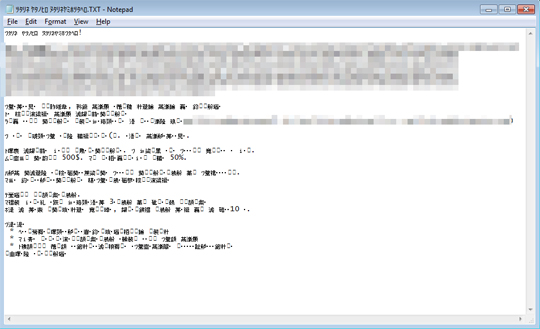## SOLUTION

Minimum Scan Engine: 9.850
FIRST VSAPI PATTERN FILE: 15.834.02
FIRST VSAPI PATTERN DATE: 28 Apr 2020
VSAPI OPR PATTERN File: 15.835.00
VSAPI OPR PATTERN Date: 29 Apr 2020

Step 1

Trend Micro Predictive Machine Learning detects and blocks malware at the first sign of its existence, before it executes on your system. When enabled, your Trend Micro product detects this malware under the following machine learning name:

• Troj.Win32.TRX.XXPE50FFF034

Step 2

Before doing any scans, Windows 7, Windows 8, Windows 8.1, and Windows 10 users must disable System Restore to allow full scanning of their computers.

Step 3

Note that not all files, folders, and registry keys and entries are installed on your computer during this malware's/spyware's/grayware's execution. This may be due to incomplete installation or other operating system conditions. If you do not find the same files/folders/registry information, please proceed to the next step.

Step 4

Restart in Safe Mode

Step 5

Delete this registry value

Important: Editing the Windows Registry incorrectly can lead to irreversible system malfunction. Please do this step only if you know how or you can ask assistance from your system administrator. Else, check this Microsoft article first before modifying your computer's registry.

Step 6

Delete this registry key

Important: Editing the Windows Registry incorrectly can lead to irreversible system malfunction. Please do this step only if you know how or you can ask assistance from your system administrator. Else, check this Microsoft article first before modifying your computer's registry. Before you could do this, you must restart in Safe Mode. For instructions on how to do this, you may refer to this page If the preceding step requires you to restart in safe mode, you may proceed to edit the system registry.

• In HKEY_CURRENT_USER\Software
• hetvuEz
• In HKEY_CURRENT_USER\Software
• {5 random characters}

Step 7

Search and delete these files